# K2 mesh deformation issues when geometry changes workplane

Greetings!
Using K2 to simulate a mesh approximation problem, I faced an issue.
Note: The constraint fix the mesh vertices in position in the XY direction and allow freedom in Z direction. While the “Angle” component helps to equalize angles.

The base mesh I created had vertices along the X axis and Y axis, this create an unexpected result (Image 1 - Starting condition, Image 2- Solution)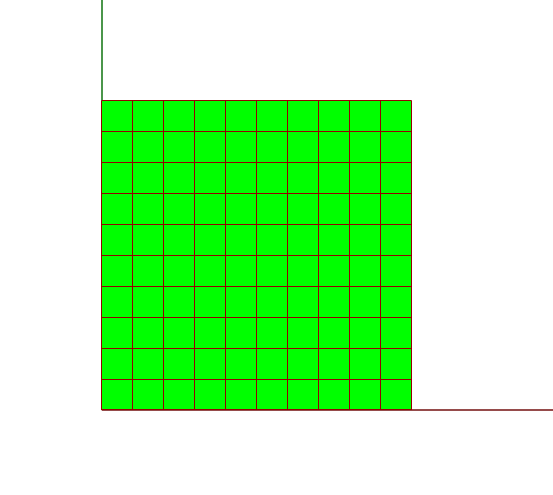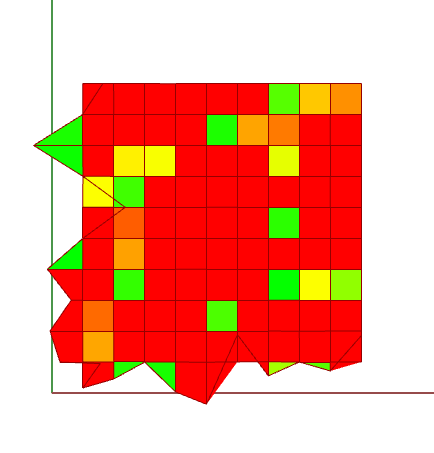I changed the position of the base mesh to have all the vertices in +X,+Y plane, this gave results that were accurate and solved the issue. (Image 5- starting position, Image 4- Solution)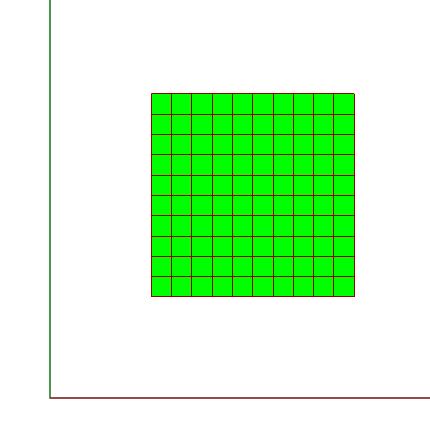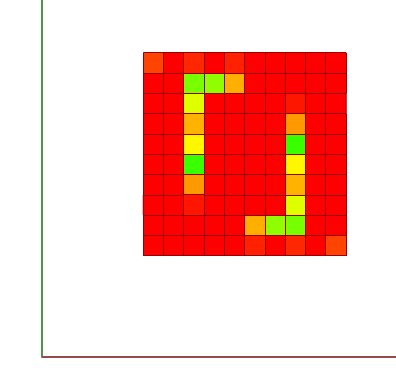This led to try another setup i.e having vertices in the X,-Y as well and the same problem occured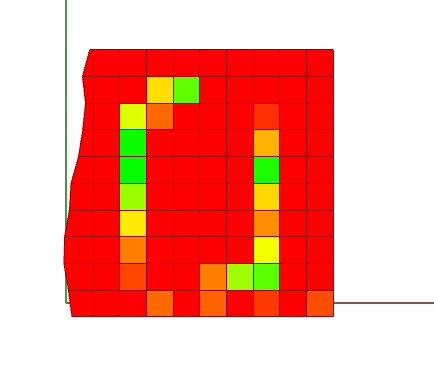The solution ideally seems to be to move all the meshes in question into the positive XYZ plane. I might be completly wrong, but is there a way to have the engine find solutions even when the mesh are existing in different 3D spaces.

What are you solving for? best to post the definition for people to be able to help.

You can set up your simulation relative to any plane, but if you have a horizontal mesh plane with points constrained to only move vertically(i.e. normal to the starting mesh), it’s going to behave differently to a vertical mesh plane with points also constrained to move vertically(i.e. tangent to the starting mesh).

Agreed, if the orientation of the starting mesh changes ie, normal along X or Y axis, then the mesh would definitly behave differently.
The problem I am trying to solve is as such, given a mesh input I want to deform the starting mesh such that it approximates the input mesh. This is being done by trying to equalize the angle between the MeshVertexNormal and MeshEdges for each vertex of the input mesh and starting mesh. (This is the first step, as I plan to add other contraints once this goal is achieved)
You can see that the solution is accurate when vertices of the starting mesh are not ‘along’ the X and Y axes but in the +X+Y plane. (Images 1 and 2)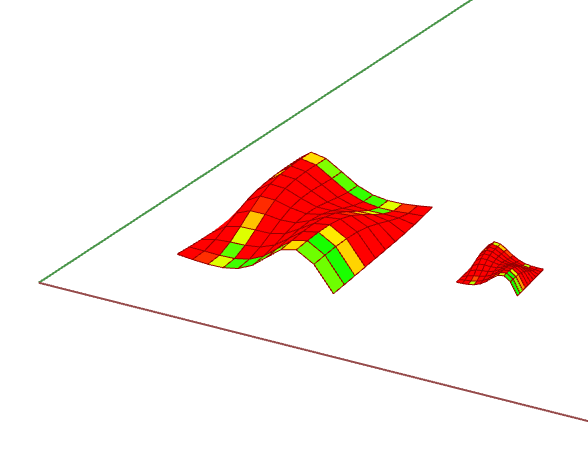The vertices behave differently when their starting condition is ON the X or Y axis.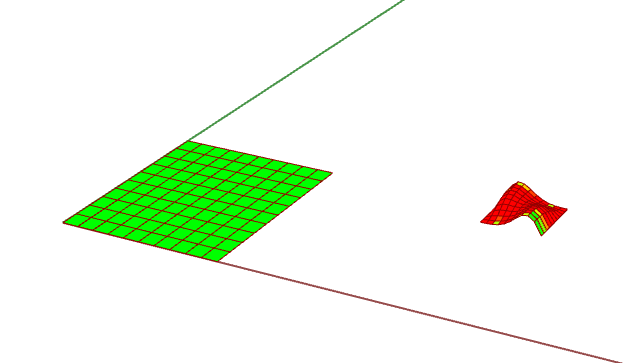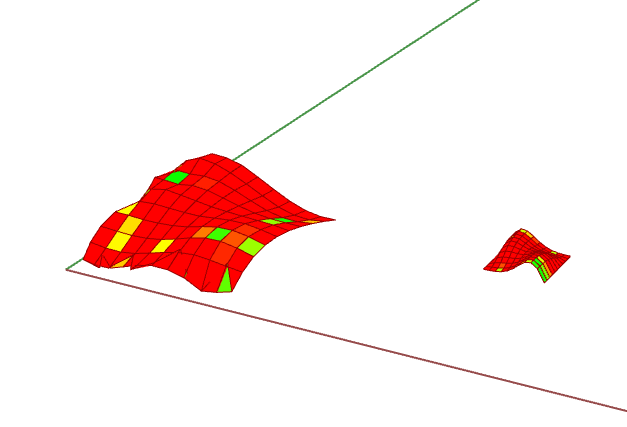The solution seems to be that the edge vertices should not be along an axis.

Without seeing the file it’s impossible to say,
I’d guess though that maybe it has to do with the the way you’re measuring your initial angles.

Agreed, I will check if the error is in the way I am measuring the initial angles.
I have shared the file here, pls share your views.
Share_Forum.gh (49.8 KB)

If the aim is to have the starting mesh match the directions of the target, here’s a simpler way:

edgedirections.gh (13.4 KB)

1 Like

Thank You for sharing this solution!!
One of my goals is to contraint the movement of the vertices in a range (ex. 0-10) on the Z axis. This will mean that the updated mesh will not have the exact form of the input mesh but something similar within the limits and the edge direction will also not be exactly equal to that of the input mesh. Is there a way to set such a boundary condition?
I did try “Clamp Length” for the vertices in Z direction in the file but does not seem to work that way.